## Differentiation

Given a function y = ƒ( x), its derivative, denoted by y′ or dy/ dx, gives the instantaneous rate of change of ƒ with repect to x. Geometrically, this gives the slope of the curve (that is, the slope of the tangent line to the curve) y = ƒ( x).Figure 1

The second derivative identifies the concavity of the curve y = ƒ( x). A portion of a differentiable curve y = ƒ( x) from x = a to x = b is said to be concave up if the curve lies above its tangent lines between a and b, and concave down if it lies below its tangent lines.

A curve y = ƒ ( x) is concave up at those points x where the second derivative is positive, and concave down where the second derivative is negative. Points where the concavity changes are called inflection points and are located at those points x 0 where ƒ n ( x 0) = 0 but ƒ m ( x 0) ≠ 0.Figure 2

Table 1  lists the most frequently used properties of derivatives and, for later reference, the corresponding properties of integrals.

TABLE 1 Computational Properties of Differentiation and IntegrationIn addition to being familiar with the definitions and fundamental properties, you should, of course, be able to actually differentiate a function. Although Table below does not contain every differentiation formula, it will probably suffice to differentiate almost every function you are likely to encounter in practice. Again, for later reference, integration formulas are listed alongside the corresponding differentiation formulas. (Note: To avoid the repetition of writing “+c” after every result in the right‐hand column, the arbitrary additive constant c has been omitted from each of the integration formulas, as in Table 1.)

TABLE 2 Differentiation and Integration FormulasExample 1: Differentiate each of the following:

a. y = 3 x 2 − 5 x + 8

b. y = x 2e x

c. y = In x/ x

d. y = ( x 3 + x − 1) 4

e.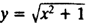f. y = sin( x 2)

g. y = sin 2 x

h. y = e tan x

i.The solutions are as follows:

a. y′ = 6 x − 5

b. Using the product rule, y′ = x 2 · e x · e x · 2 x = xe x ( x + 2)

c. By the quotient rule,All of the remaining parts use the chain rule (as embodied in the formulas in ).

d. y′ = 4( x 3 + x − 1) 3 · (3 x 2 + 1)

e.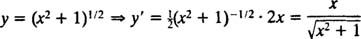f. y′ = 2 x cos( x 2)

g. y = (sin x) 2y′ = 2 sin x cos x = sin 2 x

h. y′ = e tan x sec 2 x

i.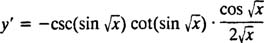Example 2: What is the equation of the tangent line to the curve y = e x In x at the point (1, 0)?

The first step is to find the slope of the tangent line at x = 1, which is the value of the derivative of y at this point:

slope at point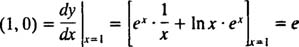Since the point‐slope formula says that the straight line with slope m which passes through the point ( x 0, y 0) has the equationthe equation of the desired tangent line is y = e( x −1).

Example 3: Is the curve y = arcsinconcave up or is it concave down at the point (?

Concavity is determined by the sign of the second derivative.

Since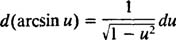the first derivative of y = arcsinisIts second derivative is therefore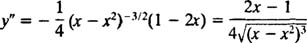For x + ¼, the denominator in the expression above for y″ is positive (as it is for any x in the interval 0 < x < 1), but the numerator is negative. Therefore, y″(¼) < 0), and the curve is concave down at the point.

Example 4: consider the curve given implicitly by the equation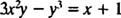What is the slope of this curve at the point where it crosses the x axis?

To find the slope of a curve defined implicitly (as is the case here), the technique of implicit differentiation is used: Differentiate both sides of the equation with respect to x; then solve the resulting equation for y′.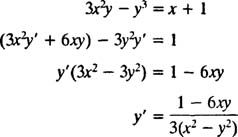The curve crosses the x axis when y = 0, and the given equation clearly implies that x = − 1 at y = 0. From the expression directly above, the slope of the curve at the point (−1, 0) is